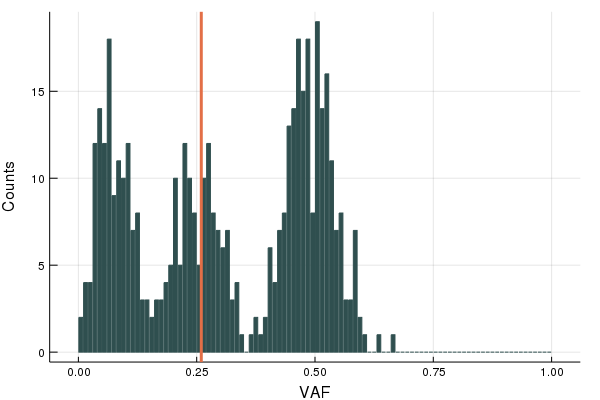dummy-link

6

3

1

1

# CancerSeqSim

Simulate tumour VAFs with different clonal structures. Package is written in the Julia programming language.

Package hosts code related to the simulation framework used in the following publications:

## Getting Started

To download the package, once you're in a Julia session type the following command:

``````using Pkg
Pkg.add("CancerSeqSim")
``````

## Example

To simulate a tumour and generate synthetic sequencing data simply invoke the `simulate` command. There are many arguments that can be changed, for example it is possible to change the mutation rate or the number of subclones introduced into the population. To see all the optional arguments you can type `?simulate` in a julia session. The command below will simulate a tumour with a single subclone with frequency between 0.4 and 0.6, mutation rate = 10 and 200 clonalmutations.

``````simdata = simulate(0.4, 0.6, μ = 10.0, clonalmutations = 200)
``````

A summary of the simulation will automatically be printed out.

A VAF histogram can be generated which uses the Plots.jl package, so you'll need to install and load the package first:

``````Pkg.add("Plots.jl")
using Plots
``````

Then using the `plot` function on a simulation object will generate the histogram, the red line shows the frequency of the subclone.

``````plot(simdata)
``````03/19/2017

5 months ago

1 commits